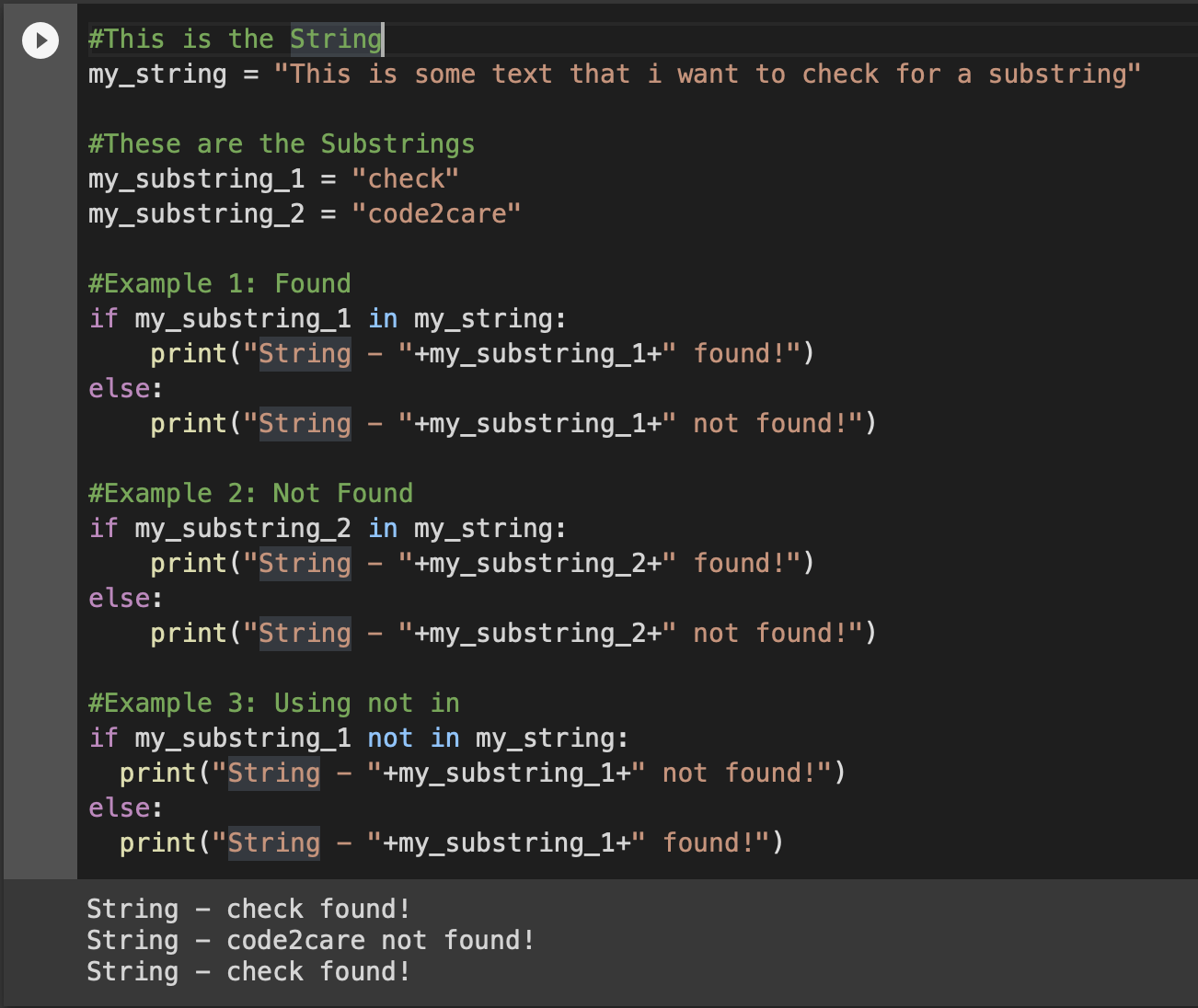# Check if String Contains a Substring - Python

In this article, we will take a look at three different ways various examples in Python to check if a string contains a substring,

1. Using the in and not in operator,
2. Using the String index() & find() methods,
3. Using Regular Expressions,

## 1. Check Substring using in/not in Operator

This is the most commonly used way in Python to check a string contains the provided substring,

Example:
``````#This is the String
my_string = "This is some text that I want to check for a substring"

#These are the Substrings
my_substring_1 = "check"
my_substring_2 = "code2care"

#Example 1: Found
if my_substring_1 in my_string:
print("String - "+my_substring_1+" found!")
else:

if my_substring_2 in my_string:
print("String - "+my_substring_2+" found!")
else:

#Example 3: Using not in
if my_substring_1 not in my_string:
else:
print("String - "+my_substring_1+" found!")``````

String - check found!
String - check found!

## 2. Check Substring using String.find() or String.index()

Note that if you use if/else with index(), if there is no match in substring you will get ValueError, so its better to use try with else,

Example: Using String.index(str)
``````#This is the String
my_string = "This is some text that I want to check for a substring"

#These are the Substrings
my_substring_1 = "check"
my_substring_2 = "code2care"

#Example 1: Found
try:
my_string.index(my_substring_1)
except ValueError:
print("String - "+my_substring_1+" found!")
else:

try:
my_string.index(my_substring_2)
except ValueError:
print("String - "+my_substring_1+" found!")
else:

if my_string.index(my_substring_2):
print("String - "+my_substring_1+" found!")
else:
Output:

String - check found!
---------------------------------------------------------------------------
ValueError Traceback (most recent call last)
<ipython-input-19-eed4a6b4d213> in <module>()
26 #Example 1: Error
27
---> 28 if my_string.index(my_substring_2):
29 print("String - "+my_substring_1+" found!")
30 else:

Example: Using String.find(str)
``````#This is the String
my_string = "This is some text that I want to check for a substring"

#These are the Substrings
my_substring_1 = "check"
my_substring_2 = "code2care"

#Example 1: Found
if my_string.find(my_substring_1) != -1:
print("String - "+my_substring_1+" found!")
else:

if my_string.find(my_substring_2) != -1:
print("String - "+my_substring_2+" found!")
else:

if my_string.find(my_substring_2) == -1:
else:
print("String - "+my_substring_2+" found!")``````

## 3. Check Substring using Regular Expressions (RegEx)

``````import re

mystring = "Today is a good day!"
substring = r"good"

matches = re.finditer(substring, mystring, re.MULTILINE)

for matchNum, match in enumerate(matches, start=1):

print ("Match {matchNum} was found at {start}-{end}: {match}".format(matchNum = matchNum, start = match.start(), end = match.end(), match = match.group()))``````

Output:

Match 1 was found at 11-15: goodPython Example - Find Substring using in Operator
-

Have Questions? Post them here!